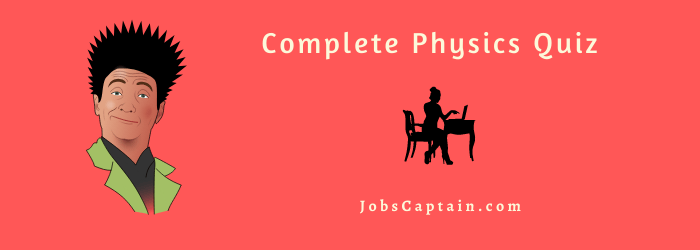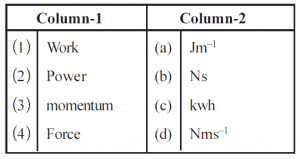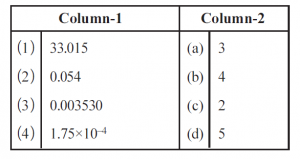# Physics Quiz OnlineThe Science and Technology Physics Questions and answers, Physics Quiz 2 is based on objective questions, which we covered measurement, dimension, and different types of physics question answers.

.

## Online Physics Quiz with Answers

Question 1: Which pair given below having a different dimension?

(A) Torque and Work

(B) Angular momentum, Plank.s constant

(C) Impulse of force & linear momentum

(D) Tension, Surface tension

(D) Tension, Surface tension

Question 2: Force acting on a body is 10N. If the unit of mass and distance become double and unit of time becomes half then the magnitude of force in the new unit system will be  ____ N.

(A) 1.6

(B) 16

(C) 160

(D) 1600

(C) 160

Question 3: Unit of power 100erg min.1, unit of time is 1 h, unit of force is 60 dyne then unit of length is _____ cm.

(A) 1

(B) 10

(C) 100

(D) 1000

(C) 100

Question 4:  When 10 N force is act on a particle momentum obtained is 1 SI, the frequency of oscillation for a particle is ____.

(A) 1 Hz

(B) 10 Hz

(C) 100 Hz

(D) 1 KHz

(B) 10 Hz

Question 5: 100 g mass, 10 cm distance, 0.1s time are taken as units in the new system. Unit of work = ______ in new system.

(A) 0.01 J

(B) 0.1 J

(C) 1 J

(D) 10 J

(B) 0.1 J

Question 6: A micrometer screw with pitch 0.5 mm and 50 divisions on the circular scale is used to measure the diameter of a sphere. The least count of micrometer screws is ______.

(A) 0.1 cm

(B) 0.01 cm

(C) 0.001 cm

(D) 0.05 cm

(C) 0.001 cm

Question 7: The diameter of a wire is measured with a micrometer of least count 0.01 mm. Reading of the main scale is 0 mm and reading of circular scale are 48 divisions then the diameter of a wire is ________.

(A) 0.48 cm

(B) 0.048 cm

(C) 0.24 cm

(D) 0.0048 cm

(B) 0.048 cm

Question 8: Match the physical quantity in column – 1 with SI unit in Column – 2.(A). 1 (c), 2 (d), 3 (b), 4 (a)

(B). 1 (b), 2 (c), 3 (a), 4 (d)

(C). 1 (d), 2 (b), 3 (c), 4 (a)

(D). 1 (c), 2 (d), 3 (a), 4 (b)

(A). 1 (c), 2 (d), 3 (b), 4 (a)

Question 9: Match the measurement in column – 1  with significant digits in column – 2.(A). 1 (b), 2 (a), 3 (d), 4 (c)

(B). 1 (d), 2 (c), 3 (b), 4 (a)

(C). 1 (d), 2 (a), 3 (c), 4 (a)

(D). 1 (b), 2 (c), 3 (d), 4 (a)

(B) 1 (d), 2 (c), 3 (b), 4 (a)

Assertion – Reason type Question:

Instruction: Read assertion and reason carefully, select a proper option from given below

1. Both assertion and reason are true and reason explains the assertion
2. Both assertion and reason are true but reason does not explain the assertion
3. Assertion is true but the reason is false
4. Assertion is false and the reason is true

Question 11 :

Assertion: Light year and wavelength both represent distance.
Reason: Both having dimensions of time.

(A) a

(B) b

(C) c

(D) d

(B) b

Question 12 :

Assertion: The distance of stars, which are further away than 100 light-years cannot measured with the method of parallax removal.
Reason: Angle of parallax removal cannot be measured accurately.

(A) a

(B) b

(C) c

(D) q

(A) a

Question 13:

Assertion: Dimension of Surface tension and Surface energy are equal.
Reason: Their SI units are equal.

(A) a

(B) b

(C) c

(D) d

(C) c

Question 14:

Assertion: y = A sin (wt . kx) and (wt . kx) is dimensionless.
Reason: The dimension of k is M°L1T°

(A) a

(B) b

(C) c

(D) d

(C) c

Question 15:

Assertion: In all measurements, the last significant digit is more inaccurate.
Reason : d = 0.9 m, d = 0.90 m and d = 0.900 m the d = 0.900 m is more accurate.

(A) a

(B) b

(C) c

(D) d